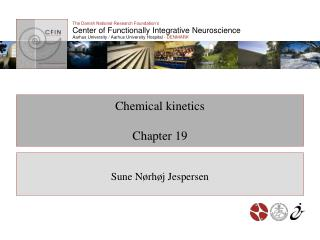# Chemical kinetics Chapter 19 - PowerPoint PPT PresentationDownload PresentationChemical kinetics Chapter 19

Chemical kinetics Chapter 19Download Presentation## Chemical kinetics Chapter 19

- - - - - - - - - - - - - - - - - - - - - - - - - - - E N D - - - - - - - - - - - - - - - - - - - - - - - - - - -
##### Presentation Transcript

1. Chemical kineticsChapter 19 Sune Nørhøj Jespersen

2. Learning objectives After working with this material, you should be able to: • Define reaction rate constants. • Write down basic differential equations for chemical kinetics, including law of mass action, and solve simple cases. • Explain the principle of detailed balance. • State the Arrhenius law

3. K A B Reaction rates Equilibrium: Kinetics: kf A B kr Rate constants: kf: Probalility of one A molecule to convert to B per unit time. kr: Same but in the opposite direction.

4. Kinetic rate equations In a short time interval dt and like wise Coupled first order differential equations

5. General solution Eigenvalues of k (in this example, one will be 0); V eigenvectors

6. General solution For example: Can be solved analytically only in simple cases for more involved reaction. Otherwise numerical solutions are valuable.

7. kf A B Example ? if is conserved

8. Detailed balance From reversibility of microscopic laws Principle of detailed balance This implies steady state Comparing to the equilibrium constant of chapter 13:

9. Example I I kAI kAI kBI kIB kBI kIA kAB B A kBA A B kBA Figure 19.1 The principle of detailed balance is satisfied by mechanism (a) between three states A,I, and B, but violated by mechanism (b). Forward rates must equal reverse rates for each pair of states.

10. Mass action Here we stipulate is proportional to the propability that a A molecules are close Number of pairs Note

11. Arrhenius depends strongly on temperature. In equilibrium: Kinetics: activation energies.

12. Arrhenius Population Transition state Reactants Products Energy